• 可以运行，有说明 有对图像DFT与DCT变换的对比与说明matlab
• 离散傅里叶变换，从网上下载，并经过改动后可以使用
•  对一张图像使用傅立叶变换就是将它分解成正弦和余弦两部分。也就是将图像从空间域(spatial domain)转换到频域(frequency domain)。 这一转换的理论基础来自于以下事实：任一函数都可以表示成无数个正弦和余弦函数...
1.1傅立叶图像
对一张图像使用傅立叶变换就是将它分解成正弦和余弦两部分。也就是将图像从空间域(spatial domain)转换到频域(frequency domain)。 这一转换的理论基础来自于以下事实：任一函数都可以表示成无数个正弦和余弦函数的和的形式。傅立叶变换就是一个用来将函数分解的工具。 2维图像的傅立叶变换可以用以下数学公式表达: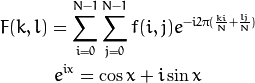式中 f 是空间域(spatial domain)值， F 则是频域(frequencydomain)值。 转换之后的频域值是复数，因此，显示傅立叶变换之后的结果需要使用实数图像(real image) 加虚数图像(complex image), 或者幅度图像(magitude image)加相位图像(phase image)。 在实际的图像处理过程中，仅仅使用了幅度图像，因为幅度图像包含了原图像的几乎所有我们需要的几何信息。然而，如果你想通过修改幅度图像或者相位图像的方法来间接修改原空间图像，你需要使用逆傅立叶变换得到修改后的空间图像，这样你就必须同时保留幅度图像和相位图像了。
在此示例中，我将展示如何计算以及显示傅立叶变换后的幅度图像。由于数字图像的离散性，像素值的取值范围也是有限的。比如在一张灰度图像中，像素灰度值一般在0到255之间。 因此，我们这里讨论的也仅仅是离散傅立叶变换(DFT)。 如果你需要得到图像中的几何结构信息，那你就要用到它了。请参考以下步骤(假设输入图像为单通道的灰度图像 I)

1.2函数
(1)int getOptimalDFTSize(int vecsize)
该函数是为了获得进行DFT计算的最佳尺寸。因为在进行DFT时，如果需要被计算的数字序列长度vecsize为2的n次幂的话，那么其运行速度是非常快的。如果不是2的n次幂，但能够分解成2,3,5的乘积，则运算速度也非常快。这里的getOptimalDFTSize()函数就是为了获得满足分解成2,3,5的最小整数尺寸。很显然，如果是多维矩阵需要进行DFT，则每一维单独用这个函数获得最佳DFT尺寸。
(2)void copyMakeBorder(InuptArray src, OutputArray dst, int top ,int bottom, int left, int right, int borderType, const Scalar&value=Scalar())
该函数是用来扩展一个图像的边界的，第3～6个参数分别为原始图像的上下左右各扩展的像素点的个数，第7个参数表示边界的类型，如果其为BORDER_CONSTANT，则扩充的边界像素值则用第8个参数来初始化。将src图像扩充边界后的结果保存在dst图像中。
(3)merge()函数是把多个但通道数组连接成1个多通道数组，而split()函数则相反，把1个多通道函数分解成多个但通道函数。
(4)void magnitude(InputArray x, InputArray y, OutPutArray magnitude)
该函数是计算输入矩阵x和y对应该的每个像素平方求和后开根号保存在输出矩阵magnitude中。
(5)函数log(InputArraysrc, OutputArray dst)是对输入矩阵src中每个像素点求log，保存在输出矩阵dst的相对应的位置上。
1.3过程描述
将图像延扩到最佳尺寸。离散傅立叶变换的运行速度与图片的尺寸息息相关。当图像的尺寸是2， 3，5的整数倍时，计算速度最快。因此，为了达到快速计算的目的，经常通过添凑新的边缘像素的方法获取最佳图像尺寸。函数 getOptimalDFTSize() 返回最佳尺寸，而函数copyMakeBorder() 填充边缘像素:

Mat padded;                            //将输入图像延扩到最佳的尺寸
int m = getOptimalDFTSize( I.rows );
int n = getOptimalDFTSize( I.cols ); // 在边缘添加0
copyMakeBorder(I, padded, 0, m - I.rows, 0, n - I.cols, BORDER_CONSTANT, Scalar::all(0));

添加的像素初始化为0.
为傅立叶变换的结果(实部和虚部)分配存储空间。傅立叶变换的结果是复数，这就是说对于每个原图像值，结果是两个图像值。此外，频域值范围远远超过空间值范围，因此至少要将频域储存在 float 格式中。结果我们将输入图像转换成浮点类型，并多加一个额外通道来储存复数部分：

Mat planes[] = {Mat_<float>(padded), Mat::zeros(padded.size(), CV_32F)};
Mat complexI;
merge(planes, 2, complexI);         // 为延扩后的图像增添一个初始化为0的通道

进行离散傅立叶变换。支持图像原地计算 (输入输出为同一图像):

dft(complexI, complexI);            // 变换结果很好的保存在原始矩阵中

将复数转换为幅度.复数包含实数部分(Re)和复数部分 (imaginary - Im)。 离散傅立叶变换的结果是复数，对应的幅度可以表示为: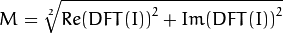转化为OpenCV代码:

split(complexI,planes);                   // planes = Re(DFT(I), planes = Im(DFT(I))
magnitude(planes, planes, planes);// planes =magnitude
Mat magI = planes;

对数尺度(logarithmicscale)缩放. 傅立叶变换的幅度值范围大到不适合在屏幕上显示。高值在屏幕上显示为白点，而低值为黑点，高低值的变化无法有效分辨。为了在屏幕上凸显出高低变化的连续性，我们可以用对数尺度来替换线性尺度: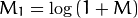转化为OpenCV代码:

magI += Scalar::all(1);                    // 转换到对数尺度
log(magI, magI);

剪切和重分布幅度图象限. 还记得我们在第一步时延扩了图像吗? 那现在是时候将新添加的像素剔除了。为了方便显示，我们也可以重新分布幅度图象限位置(注：将第五步得到的幅度图从中间划开得到四张1/4子图像，将每张子图像看成幅度图的一个象限，重新分布即将四个角点重叠到图片中心)。 这样的话原点(0,0)就位移到图像中心。

int cx = magI.cols/2;
int cy = magI.rows/2;

Mat q0(magI, Rect(0, 0, cx, cy));   // Top-Left - 为每一个象限创建ROI
Mat q1(magI, Rect(cx, 0, cx, cy));  // Top-Right
Mat q2(magI, Rect(0, cy, cx, cy));  // Bottom-Left
Mat q3(magI, Rect(cx, cy, cx, cy)); //Bottom-Right

Mat tmp;                           // 交换象限 (Top-Left with Bottom-Right)
q0.copyTo(tmp);
q3.copyTo(q0);
tmp.copyTo(q3);

q1.copyTo(tmp);                    // 交换象限 (Top-Right with Bottom-Left)
q2.copyTo(q1);
tmp.copyTo(q2)

7.归一化. 这一步的目的仍然是为了显示。 现在我们有了重分布后的幅度图，但是幅度值仍然超过可显示范围[0,1] 。我们使用 normalize()函数将幅度归一化到可显示范围。

normalize(magI, magI, 0, 1, CV_MINMAX); // 将float类型的矩阵转换到可显示图像范围
// (float [0， 1]).

1.4完整程序

#include "opencv2/core/core.hpp"
#include "opencv2/imgproc/imgproc.hpp"
#include "opencv2/highgui/highgui.hpp"
#include <iostream>
#include <fstream>
using namespace cv;
using namespace std;

int main(int argc, char ** argv)
{
//以灰度图像模式读入原始图像
const char* filename ="4.jpg";
if( I.empty())
return -1;

//将图像扩展到最佳尺寸,边界用0补充
Mat padded;                            //expand input image to optimal size
//将添加的像素初始化为0
int m = getOptimalDFTSize( I.rows );//计算最佳扩充尺寸
int n = getOptimalDFTSize( I.cols ); // on the border add zero values
copyMakeBorder(I, padded, 0, m - I.rows, 0, n - I.cols, BORDER_CONSTANT, Scalar::all(0));		//扩充图像

//为傅里叶变换的结果(实部和虚部)分配存储空间
//将planes数组组合合并成一个多通道的数组complexI
Mat complexI;
merge(planes, 2, complexI);         // Add to the expanded another plane with zeros	//合并通道

//进行傅里叶变换
dft(complexI, complexI);            // this way the result may fit in the source matrix	//离散傅立叶变换

//将复数转换为幅值，即=> log(1 + sqrt(Re(DFT(I))^2 + Im(DFT(I))^2))
// compute the magnitude and switch to logarithmic scale
// => log(1 + sqrt(Re(DFT(I))^2 + Im(DFT(I))^2))
split(complexI, planes);                   // planes = Re(DFT(I), planes = Im(DFT(I))	//将存储在complexI的结果分解到planes,planes中	// 将多通道数组complexI分离成几个单通道数组，planes = Re(DFT(I), planes = Im(DFT(I))
magnitude(planes, planes, planes);// planes = magnitude		//计算复制
Mat magI = planes;

//进行对数尺度缩放
magI += Scalar::all(1);                    // switch to logarithmic scale	//用对数表示
log(magI, magI);		//求自然对数

//剪切和充分不幅度图象限
//若有奇数行或者奇数列，进行频谱裁剪
// crop the spectrum, if it has an odd number of rows or columns
//按位与，x & -2大概意思就是取不大于x 的最大偶数
magI = magI(Rect(0, 0, magI.cols & -2, magI.rows & -2));

//重新排列傅里叶图像中的象限，使得原点位于图像的中心
// rearrange the quadrants of Fourier image  so that the origin is at the image center
int cx = magI.cols/2;
int cy = magI.rows/2;

Mat q0(magI, Rect(0, 0, cx, cy));   // Top-Left - Create a ROI per quadrant
Mat q1(magI, Rect(cx, 0, cx, cy));  // Top-Right
Mat q2(magI, Rect(0, cy, cx, cy));  // Bottom-Left
Mat q3(magI, Rect(cx, cy, cx, cy)); // Bottom-Right
//交换象限(左上与右下)
Mat tmp;                           // swap quadrants (Top-Left with Bottom-Right)
q0.copyTo(tmp);
q3.copyTo(q0);
tmp.copyTo(q3);
//交换象限(右上与左下）
q1.copyTo(tmp);                    // swap quadrant (Top-Right with Bottom-Left)
q2.copyTo(q1);
tmp.copyTo(q2);

//归一化
normalize(magI, magI, 0, 1, CV_MINMAX); // Transform the matrix with float values into a
// viewable image form (float between values 0 and 1).

Mat invDFT,invDFTcvt;
idft(complexI, invDFT, DFT_SCALE | DFT_REAL_OUTPUT );//离散傅立叶逆变换
invDFT.convertTo(invDFTcvt, CV_8U);
imshow("invDFTcvt", invDFTcvt);

imshow("Input Image"       , I   );    // Show the result
imshow("spectrum magnitude", magI);
waitKey();

return 0;
}


展开全文opencv
• 关于 离散傅里叶变换 DFT 以及 快速傅里叶变换 FFT 的算法可以在 算法导论第30章 或者 其他人的博客里看到。这里不做赘述。我们来看一种有趣的函数式的实现…
关于 离散傅里叶变换 DFT 以及 快速傅里叶变换 FFT 的算法可以在 算法导论第30章 或者 其他人的博客 里看到。这里不做赘述。
我们来看一种函数式的实现：
依赖
复数类
首先，建立一个复数方法类：
// complex methods

// 构造函数，默认为 0
complex = (x, y) => { return { x: x || 0, y: y || 0 }; };
// 从弧度构造单位复数
complex.fromAngle = (angle) => complex(Math.cos(angle), Math.sin(angle));
// 复数加复数
complex.add = (a, b) => complex(a.x + b.x, a.y + b.y);
// 复数乘复数
complex.mul = (a, b) => complex(a.x * b.x - a.y * b.y, a.x * b.y + a.y * b.x);
// 数乘复数
complex.numMul = (n, c) => complex(n * c.x, n * c.y);
// 复数减复数
complex.minus = (a, b) => complex(a.x - b.x, a.y - b.y);
注意，这个复数乘复数与复数减复数是冗余的：
complex.numMul = (n, c) => complex.mul(complex(n), c);
complex.minus = (a, b) => complex.add(a, complex.numMul(-1, b));
列表重构
关于列表重构，可以参考我的另一篇博文：JavaScript 列表重构
// array restructuring
flatten = (matrix) => matrix.reduce((pre, cur) => pre.concat(cur), []);
partition = (array, n) => array.reduce((pre, cur, i) => (pre[i % n] = pre[i % n] || []).push(cur) && pre, []);
transpose = (matrix) => partition(flatten(matrix), matrix.length);
DFT & DFT的逆
这里先很不要脸地给出一行形式的代码
recursiveDFT = (a, inverse) => a.length == 1 ? a : flatten(transpose(transpose(a.reduce((pre, cur, i) => pre[i & 1].push(cur) && pre, [[], []]).map(v => recursiveDFT(v))).map((v, i) => [complex.add(v, complex.mul(complex.fromAngle(i * (inverse ? -2 * Math.PI / a.length : 2 * Math.PI / a.length)), v)), complex.minus(v, complex.mul(complex.fromAngle(i * (inverse ? -2 * Math.PI / a.length : 2 * Math.PI / a.length)), v))]))).map(v => inverse ? complex.numMul(1 / a.length, v) : v);
传参
目前这个函数尚不类型安全，必须要有严格的传参方式才能保证不出错。
第一个参数是一个数组：
其中每个元素都要有 x 与 y 这两个属性。数组的长度是2的幂（1, 2, 4, 8, 16, …）
第二个参数是可判定真假的值，由于存在强制转换因此不会有问题。  当第二个参数为true时，执行DFT的逆，默认为false。
测试
由于使用了ES6的语法，浏览器的支持度查询：Arrow Functions Supported
如果你的浏览器支持，你可以尽情测试：
var x = [complex(1), complex(2), complex(0), complex(0)]; // 1 + 2x
var y = [complex(3), complex(4), complex(0), complex(0)]; // 3 + 4x

var rx = recursiveDFT(x);
recursiveDFT(rx, true); // almost same as x

var ry = recursiveDFT(y);
recursiveDFT(ry, true); // almost same as y

var rc = rx.map((v, i) => complex.mul(v, ry[i]));
recursiveDFT(rc, true); // almost same as [complex(3), complex(10), complex(8), complex(0)]

感兴趣的读者可以自行将那一行代码婊开看逻辑。
虽然我定义了几个函数(complex方法类、restructuring方法类)来处理这个问题，但是不难发现这些函数依然可以inline化组合到一起，写出一个不带分号的 One Line DFT。
展开全文javascript FFT
• DTFT DFT ZT 这些变换之间有什么联系和区别
CTFT DTFT DFT ZT 这些变换之间有什么联系和区别

CTFT是continue time fourier transform,连续时间傅里叶变换。DTFT是 discret time fourier transform，离散时间傅里叶变换。DFT　是　discret fourier transform，离散傅里叶变换,ZT是z 变换。

将CTFT的时域离散即可的DTFT，将DTFT的频域离散即可的DFT。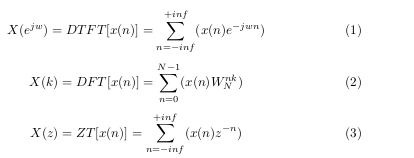展开全文• 4.知识点-傅里叶变换DFT、小波变换、高低通滤波器龙熙视觉.docx
• ## DCT变换和DFT变换

千次阅读 2018-12-16 16:45:44
DCT变换和DFT变换 1：DFT变换 这里我只谈论二维的DFT变换，有关一维的DFT，请查看一下博客，里面有详细介绍： https://blog.csdn.net/IMWTJ123/article/details/79831242 根据上面的公式，我们可以得到对应的...
DCT变换和DFT变换
1：DFT变换
这里我只谈论二维的DFT变换，有关一维的DFT，请查看一下博客，里面有详细介绍：
https://blog.csdn.net/IMWTJ123/article/details/79831242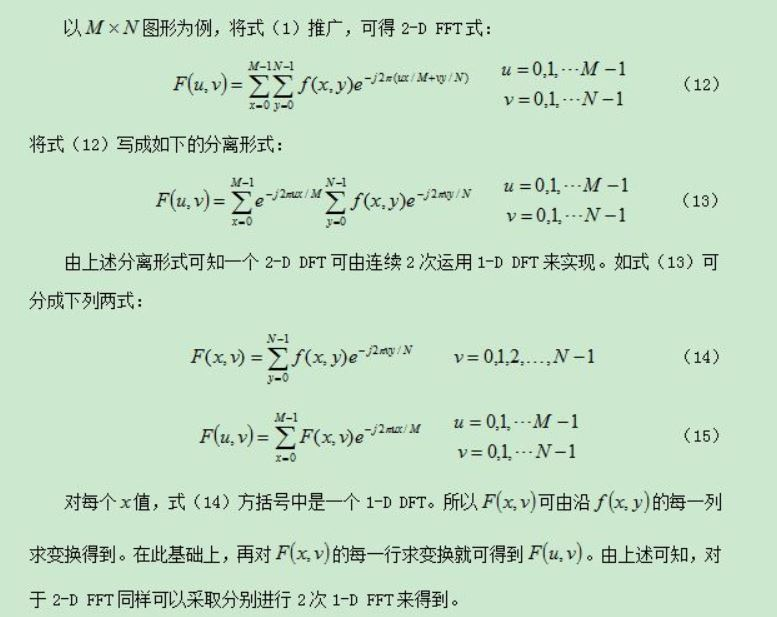根据上面的公式，我们可以得到对应的离散傅里叶变换呃代码，下面是matlab版本的代码，和公式(12)是一一对应的，时间复杂度为（MN)^2，通常记成N^4，因此对于一个512*512的图片来说，计算量就已经很大了，更不用说别的高分辨率图像了，下面的代码输入block_struct是为了方便做blockproc，如果直接将图像的值赋给f就可以直接做DFT：
function F =  dft2(block_struct)

f=block_struct.data;
[M, N] = size(f);
F = zeros(M,N);

for k = 0:M-1
for l = 0:N-1
F(k+1,l+1) = dft_atomic_sum(f,k,l,M,N);
end
end

%% computing a core of DFT2
function F_kl = dft_atomic_sum(f,k,l,M,N)

F_kl = 0;

for m=0:M-1
for n=0:N-1
F_kl = F_kl + f(m+1,n+1)*exp(-i*2*pi*( k*m/M   + l*n/N) );
end
end


因此，常用的方法就是先将图像划分为8*8的块，然后对每个块执行DFT变换：
fun_dft = @(block_struct) dft2(block_struct);
after_DFT=blockproc(Y,[8,8],fun_dft);
这样的时间复杂度就降低了很多，变成了O（8*8*block_num），其中block_num就是块的个数，因此现在常用的FFT算法，该算法的介绍很多，本文主要介绍原始DFT，对FFT感兴趣的可以查阅相关资料。
2：IDFT
IDFT为离散傅里叶变换的逆变换，公式如下;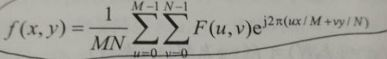相应的代码如下，是根据DFT2改编的：
function F =  idft2(block_struct)

f=block_struct.data;
[M, N] = size(f);
F = zeros(M,N);

for k = 0:M-1
for l = 0:N-1
F(k+1,l+1) = idft_atomic_sum(f,k,l,M,N);
end
end

%% computing a core of DFT2
function F_kl = idft_atomic_sum(f,k,l,M,N)

F_kl = 0;

for m=0:M-1
for n=0:N-1
F_kl = F_kl + f(m+1,n+1)*exp(i*2*pi*(k*m/M+l*n/N));
end
end

F_kl = 1/N * 1/M * F_kl ;


同样需要对图像的8*8的块进行IDFT，代码如下：
fun_idft=@(block_struct) idft2(block_struct);
after_iDFT=blockproc(after_DFT,[8,8],fun_idft);
after_iDFT=abs(after_iDFT);
可以试验一下，先进行DFT，然后进行IDFT，可以无损的恢复回来：
img=magic(8)
block_struct.data=img
after_dft=dft2(block_struct)
after_inverse_dft=abs(idft2(after_dft))
after_inverse_dft==img


3: DCT
同样的，我只对二维的DCT变换做一些介绍，一维的DCT以及相应理论可以查看下面这篇论文：
https://blog.csdn.net/qq_20613513/article/details/78744101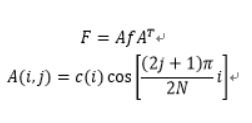产生DCT变换矩阵A的代码如下：
N=8;
A=zeros(N);
for i=0:N-1
for j=0:N-1
if i==0
a=sqrt(1/N);
else
a=sqrt(2/N);
end
A(i+1,j+1)=a*cos(pi*(2*j+1)*i/(2*N));
end
end
同理，对8*8块进行DCT变换的代码如下，如果不想使用8*8分块进行DCT，那么需要产生一个N*N大小的DCT变换矩阵A，然后使用F=AfA'计算：
fun = @(block_struct) A*(block_struct.data)*A';
after_DCT=blockproc(image,[8,8],fun);
4：IDCT
离散余弦变换的逆变换，逆变换非常简单，只需要令f=A'FA就可以了：
fun2 = @(block_struct) A'*(block_struct.data)*A;
inverse_DCT=blockproc(after_zigzag,[8,8],fun2);
5：实验结果
中间的DCT结果是我使用zigzag保留前16个系数，其余都置零得到的结果：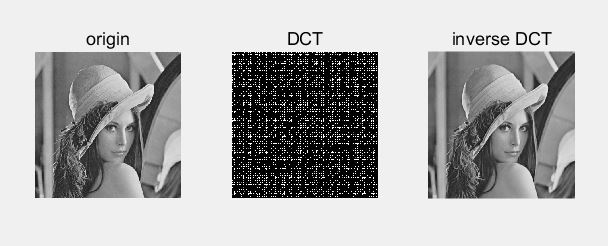下面是使用DFT变换之后的结果，第二幅图是使用abs(after_dft)得到的频谱图，第三幅图是使用angle(after_dft)得到的相位图，IDFT是逆变换之后的得到的恢复的图像。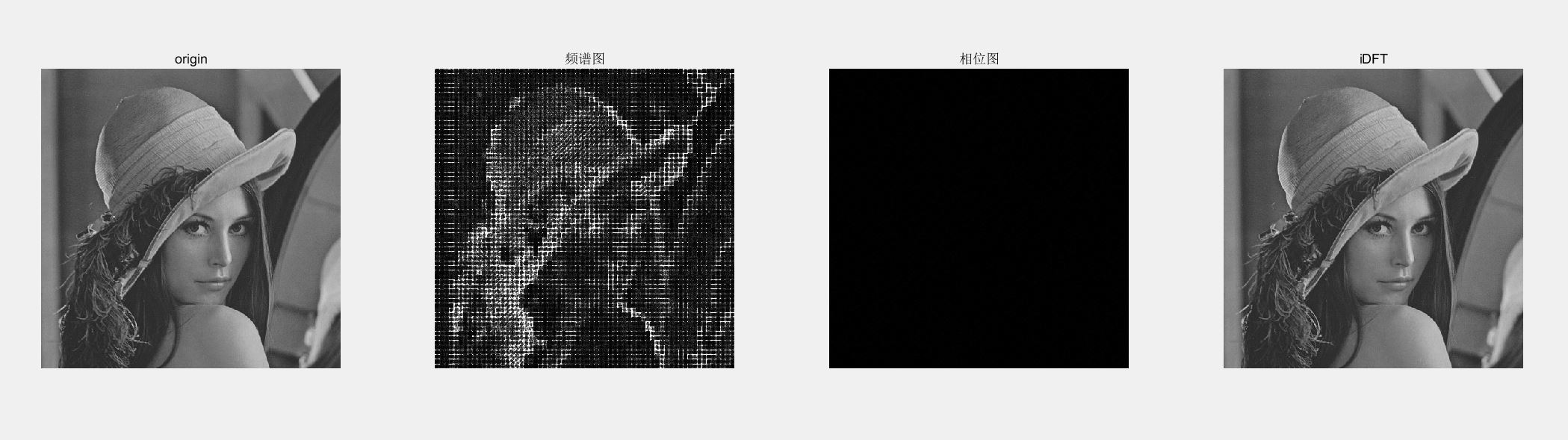展开全文IDFT JPEG
• c#写的实现DFT和FFT变换的程序.很很好的对实序列进行变换
• 目的：对图像进行离散傅立叶变换(DFT)、离散余弦变换(DCT)变换，并对图像傅立叶变换的结果进行必要解释，使用IDFT、IDCT对图像进行重建，分析重建后的图像。操作过程中获取两帧图像，对这两帧图像分别进行 DFT、DCT...
• DFT是傅里叶变换的离散形式，也即将x(t)进行傅里叶变换后进行离散采样得的函数X[jw] 傅里叶变换仅仅是抄对其进行e(jwt)的变换操作，而拉普拉斯变换则是对e(st)的操作，两者不同在于傅里叶变换是拉普拉斯变换的特殊...傅立叶分析
• 根据信号的不同类型，可以把傅立叶变换分为四类：1) 非周期性连续信号： 傅立叶变换(Fourier Transform，FT)2) 周期性连续信号： 傅立叶级数(Fourier Series，FS) 3) 非周期性离散信号： 离散时域傅立叶变换...
• C#写的信号分析类，包含DFT，AR变换，共振解调，频域特征值计算等多种算法
• 该篇包括三部分，1）引言、2）图像变化技术...为了使水印信息有更好的鲁棒性（抵抗攻击的能力）众多学者采用变换域中的嵌入方法，接下来将分别介绍基于DCT、DFT、DWT三种基础图像变换技术的数字水印方法及代码实现。计算机视觉 人工智能 数字信号处理
• MATLAB实验实现FFT DFT傅里叶变换，简单入门实验，适用小白
• function [Xk] = dft(xn,N) %computes discrete fourier transform %--------------------------------------------- %[Xk] = dft(xn,N) %Xk = DFT coeff. array over 0<= k<=N-1 %xn = one period of periodi...
• IDFT函数的计算结果与matlab的ifft（X）函数计算结果完全相同，运行速度超级快，文件包含使用示例代码和说明，写的很详细了，保证你看了就会用。
• 傅里叶变换(DFT) 首先来看看傅里叶(DFT)变换的公式 (1) FP=\frac {1}{N}\sum_{x=0}^{N-1}\sum_{y=0}^{N-1}P_{x,y}\exp(-j(\frac{2 \pi}{N})(ux+vy)) 幅度 (2) w=\sqrt{u^2+v^2} 其中 u,v代表空间频率，即灰度梯度，...
• 基于DCT变换和DFT变换的数字图像压缩Matlab仿真 分为DCT和DFT两个文件夹 matlab仿真
• opencv+pyqt5+matpoltlib实现图像变换DFT）的代码 opencv+pyqt5+matpoltlib实现图像变换DFT）的代码
• 数字信号DFT变换方法，对时域信号进行频域分析，很有帮助
• DFT:数字电路(fpga/asic)设计入门之可测试设计与可测性分析,离散傅里叶变换,(DFT)Direct Fouriet Transformer 可测试性技术（Design For Testability-DFT）就是试图增加电路中信号的可控制性和可观测性，以便及时...测试 存储 算法
• 用于实现快速傅里叶变换的matlab程序，使用时将信号x赋一初值，就能得到其变换后的信号...